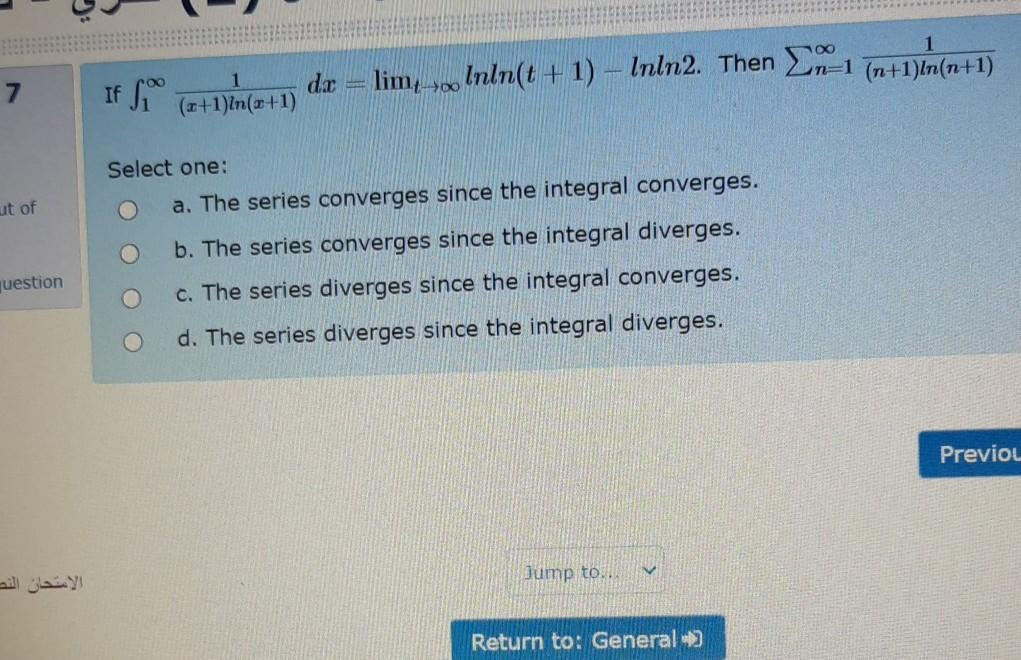### Create an Account

Home / Questions / 7 de If foo 1 (+1)In(x+1) limsoo lnln(t+1) - Inln2. Then 21 n=1 (n+1)In(n+1) ut of Select ...

# 7 de If foo 1 (+1)In(x+1) limsoo lnln(t+1) - Inln2. Then 21 n=1 (n+1)In(n+1) ut of Select one: a. The series converges since the integral converges. b. The series converges since the integral diverges

7 de If foo 1 (+1)In(x+1) limsoo lnln(t+1) - Inln2. Then 21 n=1 (n+1)In(n+1) ut of Select one: a. The series converges since the integral converges. b. The series converges since the integral diverges. O c. The series diverges since the integral converges. d. The series diverges since the integral diverges. question Previou الامتحان النص Jump to.. Return to: General)Apr 24 2021 View more View LessSubscribe To Get Solution